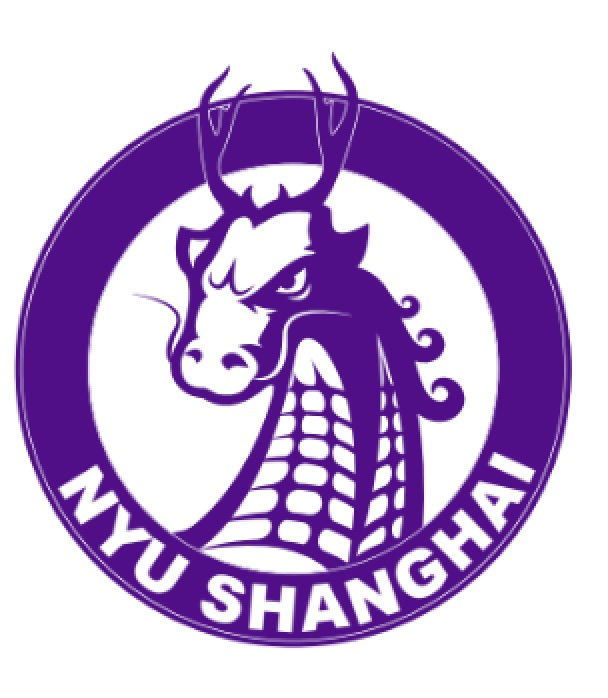# Siran LiSiran Li
Visiting Assistant Professor of Mathematics
Email
sl4025@nyu.edu

Siran Li is a Visiting Assistant Professor of Mathematics at NYU Shanghai. Siran's research interests lie in PDE (Partial Differential Equation), calculus of variations, global and geometric analysis, and applied math.

From 2009 to 2013, Siran studied math (BA) at Columbia University in NY and then obtained a DPhil from the University of Oxford, UK in 2017 under the supervision of Prof. Gui-Qiang G. Chen. Before joining NYU Shanghai, Siran was a G. C. Evans Instructor at Rice University (Houston) mentored by Prof. Robert M. Hardt, and a CRM--ISM postdoctoral fellow at McGill University (Montreal).

### Select Publications

• S. Li, On the existence of \$C^{1,1}\$-isometric immersions of several classes of negatively curved surfaces into \$\mathbb{R}^3\$, \textbf{Arch. Ration. Mech. Anal.} 236 (2020),  419--449
• S. Li, A remark on the non-compactness of \$W^{2,d}\$-immersions of \$d\$-dimensional hypersurfaces, \textbf{Proc. Amer. Math. Soc.} 148 (2020), 2245--2255
• S. Li, The Weyl problem of isometric immersions revisited, accepted by \textbf{Bulletin London Math. Soc.} (2020); Arxiv Preprint: 2004.05532
• A. Archarya, G.-Q. Chen, S. Li, M. Slemrod, and D. Wang, Fluids, elasticity, geometry, and the existence of wrinkled solutions, \textbf{Arch. Ration. Mech. Anal.} 226 (2017), 1009--1060
• G.-Q. Chen and S. Li, Global weak rigidity of the Gauss--Codazzi--Ricci equations and isometric immersions of Riemannian manifolds with lower regularity, \textbf{J. Geom. Anal.} 28 (2018), 1957--2007

### Education

• DPhil, Mathematics
University of Oxford, 2013 - 2017
• BA, Mathematics
Columbia University, 2009 - 2013

## Research Interests

• Mathematical Analysis and PDE
• Geometric Analysis
• Calculus of Variations
• Fluid and Continuum Mechanics
• Applied Mathematics